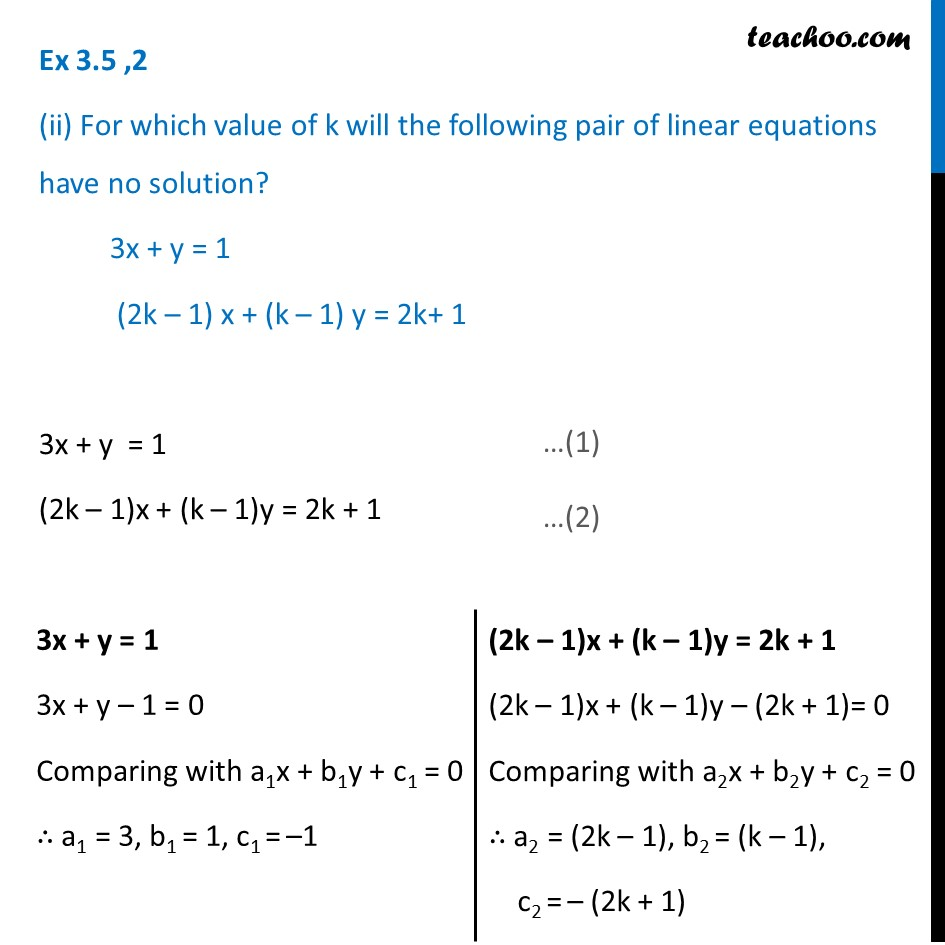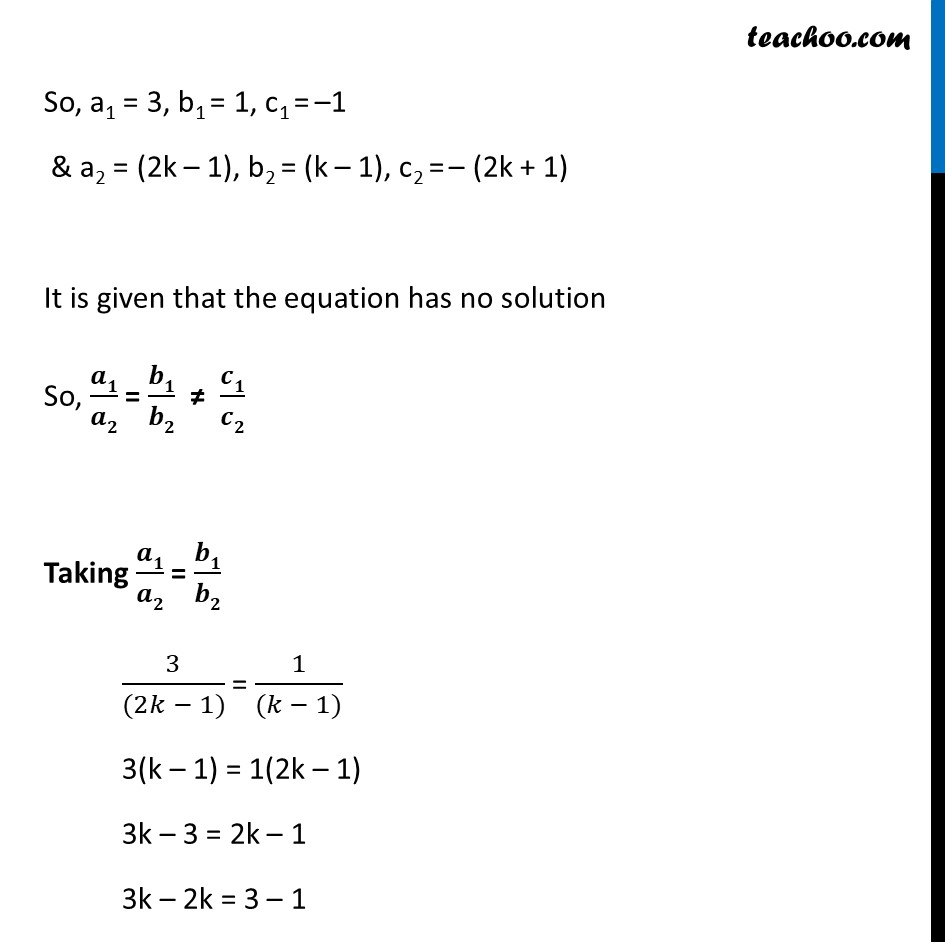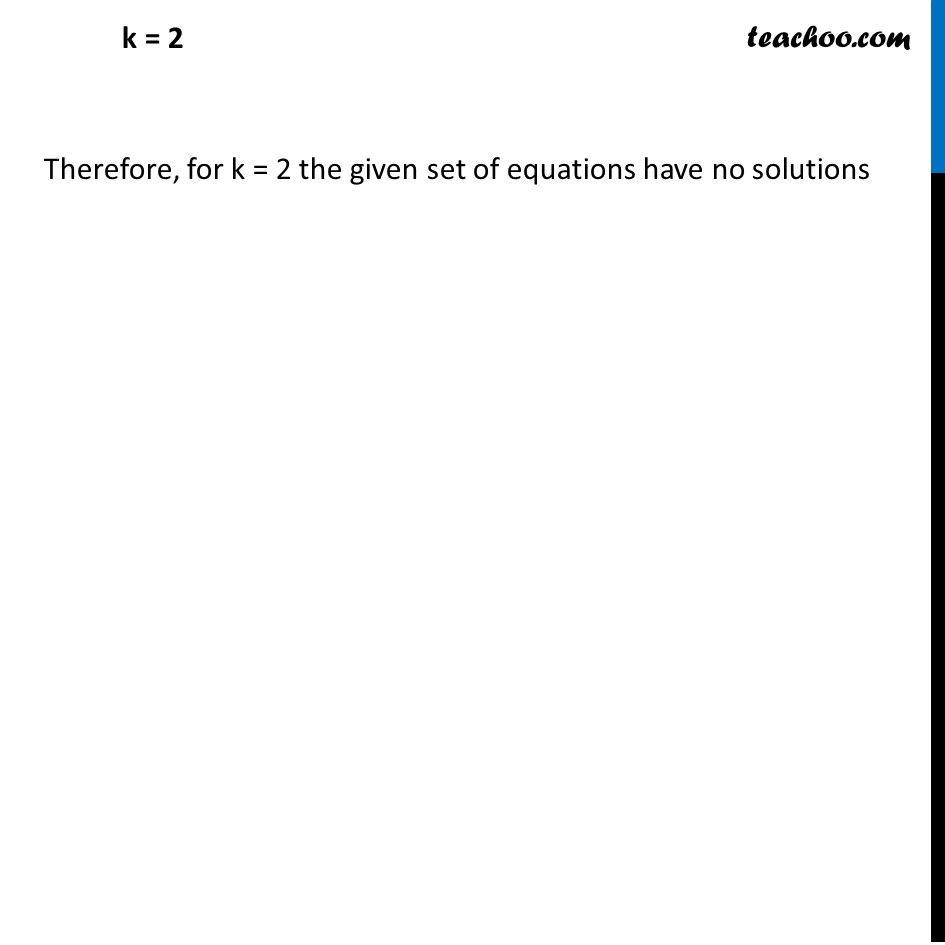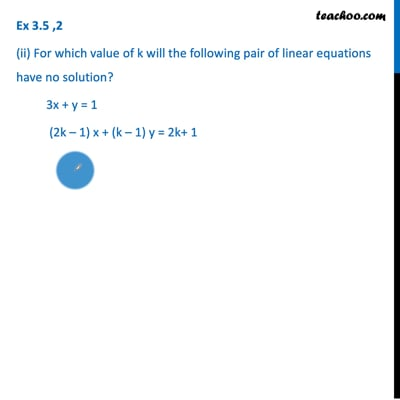Ex 3.5

Chapter 3 Class 10 Pair of Linear Equations in Two Variables
Serial order wiseThis video is only available for Teachoo black users

Introducing your new favourite teacher - Teachoo Black, at only ₹83 per month

### Transcript

Ex 3.5 ,2 (ii) For which value of k will the following pair of linear equations have no solution? 3x + y = 1 (2k – 1) x + (k – 1) y = 2k+ 1 3x + y = 1 (2k – 1)x + (k – 1)y = 2k + 1 3x + y = 1 3x + y – 1 = 0 Comparing with a1x + b1y + c1 = 0 ∴ a1 = 3, b1 = 1, c1 = –1 (2k – 1)x + (k – 1)y = 2k + 1 (2k – 1)x + (k – 1)y – (2k + 1)= 0 Comparing with a2x + b2y + c2 = 0 ∴ a2 = (2k – 1), b2 = (k – 1), c2 = – (2k + 1) So, a1 = 3, b1 = 1, c1 = –1 & a2 = (2k – 1), b2 = (k – 1), c2 = – (2k + 1) It is given that the equation has no solution So, 𝒂𝟏/𝒂𝟐 = 𝒃𝟏/𝒃𝟐 ≠ 𝒄𝟏/𝒄𝟐 Taking 𝒂𝟏/𝒂𝟐 = 𝒃𝟏/𝒃𝟐 3/((2𝑘 − 1)) = 1/((𝑘 − 1)) 3(k – 1) = 1(2k – 1) 3k – 3 = 2k – 1 3k – 2k = 3 – 1 k = 2 Therefore, for k = 2 the given set of equations have no solutions